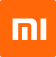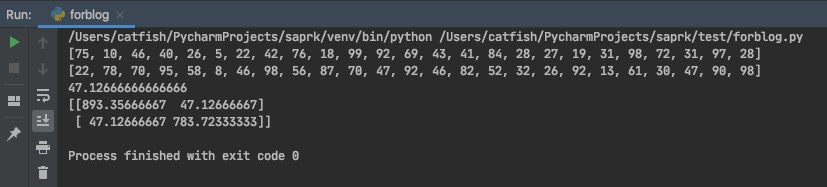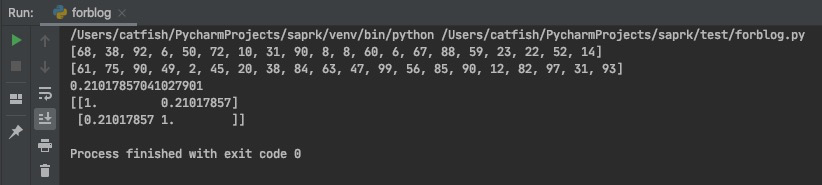2020-05-24

# 统计建模初探 —— Analysis of Correlation

[作者简介] 焦家耀，小米信息技术部售后组

## 相关性分析方法

• 基于图表的肉眼观测
• 协方差｜协方差矩阵
• 相关系数（Pearson 系数）
• 一元回归｜多元回归

### 协方差｜协方差矩阵

#### 协方差定义

$$Var(X+Y) = Var(X) + Var(Y) + 2E[(X-E(X))(Y-E(Y))]$$

$$cov(X, Y) = E{[X-E(X)][Y-E(Y)]}$$

#### 协方差矩阵定义

$$c_ij = cov(X_i, X_j) = E{[X_i-E(X_i)][X_j-E(X_j)]} \quad i, j = 1, 2, …, n$$

$$C = \begin{bmatrix}c_{11} & c_{12} & … & c_{1n} \\ c_{21} & c_{22} & … & c_{2n} \\ \vdots & \vdots & \vdots & \vdots \\ c_{n1} & c_{n2} & … & c_{nn}\end{bmatrix}$$

#### 协方差｜协方差矩阵应用### 相关系数

#### 相关系数（Pearson 系数）定义

$$\rho_{XY}=\frac{cov(X, Y)}{\sqrt{Var(X)Var(Y)}}$$

#### 相关系数的性质

• $|p_{XY}|\leq1$
• $|p_{XY}|=1$的充要条件是存在常数$a$, $b$，使得$P\{Y=aX+b\} = 1$

性质 1 可知，相关系数定量的描述了随机变量$X$, $Y$的相关程度，即$|p_{XY}|$越大，相关程度越大，$|p_{XY}|=0$相关程度最低。
性质 2 可知，$X$, $Y$完全相关是指在概率为 1 的情况下存在线性关系，于是$|p_{XY}|$也可以表示$X$, $Y$之间的线性关系紧密程度的量，当$|p_{XY}|$较大的时候，通常可以说$X$, $Y$之间线性相关程度较好。

#### 相关系数应用#### 总结

Pearson 系数在使用中经常会和斯皮尔曼相关性系数（Spearman 系数），肯德尔相关性系数（Kendall 系数）一起出现，但是三者侧重点各不相同，感兴趣的同学可以自己搜索了解一下。笔者推荐一个相关资料 三大系数

### 一元回归｜多元回归

#### 一元回归的拟合优度

$$R^2=1-\frac{\sum_i{(\hat{y}_i - y_i)^2}}{\sum_i{(\overline{y}_i - y_i)^2}} = 1 - \frac{MSE}{Var}$$

## 下集预告

Regression Analysis （回归分析）